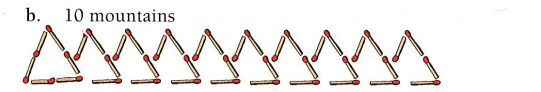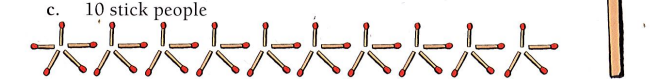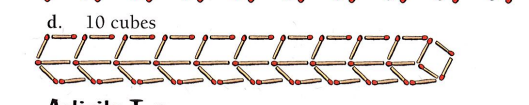## Friday, 14 October 2016

### Algebra Maths #3A 31 sticks : 3M + 1 =
10
10 x 3 = 30 30 + 1 =31B 42 sticks : - 2  4x + 2
10
10 x 4 = 40 so 40 + 2 = 42C 41 sticks : -1 4b + 1
10
10 x 4 = 40 so 40 + 1 = 41D 54 sticks : - 4 5c + 4
10
10 x 5 = 50 so 50 + 4 = 54

_____________________

1. 62 sticks         b. 84 sticks

c. 82 sticks          d. 108 sticks

_____________________

Helloo bloggers Today I had done a maths activitie. This activitie was related on Algebra. Algebra is Type of Mathematics which contains letters and other symbols, it is more like a variable. Hope you enjoy leave a comment if like it. Don't go Changing !!